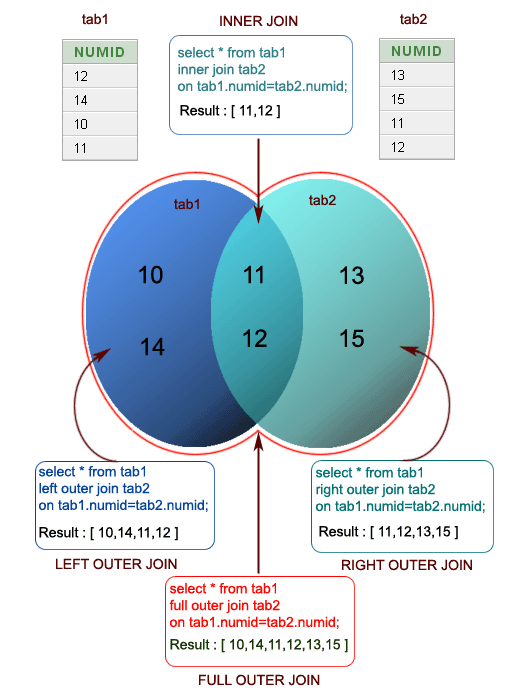﻿ R Programming: Create inner, outer, left, right join from given two data frames - w3resource# R Programming: Create inner, outer, left, right join from given two data frames

## R Programming: Data frame Exercise-13 with Solution

Write a R program to create inner, outer, left, right join(merge) from given two data frames.

Sample Solution :

R Programming Code :

``````df1 = data.frame(numid = c(12, 14, 10, 11))
df2 = data.frame(numid = c(13, 15, 11, 12))
print("Left outer Join:")
result = merge(df1, df2, by = "numid", all.x = TRUE)
print(result)
print("Right outer Join:")
result = merge(df1, df2, by = "numid", all.y = TRUE)
print(result)
print("Outer Join:")
result = merge(df1, df2, by = "numid", all = TRUE)
print(result)
print("Cross Join:")
result = merge(df1, df2, by = NULL)
print(result)
``````

Pictorial Presentation :Sample Output:

``` "Left outer Join:"
numid
1    10
2    11
3    12
4    14
 "Right outer Join:"
numid
1    11
2    12
3    13
4    15
 "Outer Join:"
numid
1    10
2    11
3    12
4    13
5    14
6    15
 "Cross Join:"
numid.x numid.y
1       12      13
2       14      13
3       10      13
4       11      13
5       12      15
6       14      15
7       10      15
8       11      15
9       12      11
10      14      11
11      10      11
12      11      11
13      12      12
14      14      12
15      10      12
16      11      12
```

R Programming Code Editor:

Have another way to solve this solution? Contribute your code (and comments) through Disqus.

Test your Programming skills with w3resource's quiz.

What is the difficulty level of this exercise?

﻿